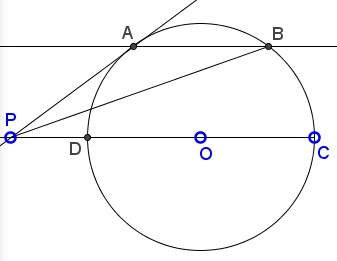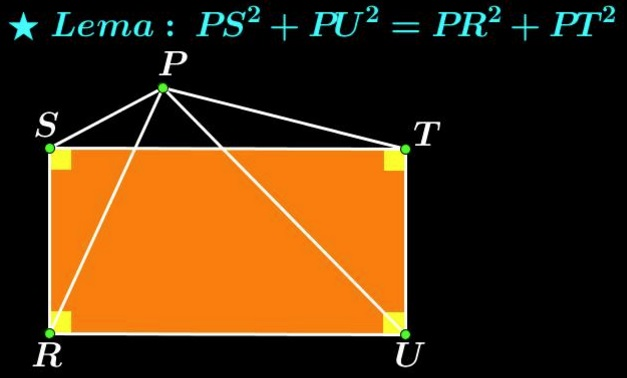# Sums of Squares in Circle

### Source

Leo Giugiuc has kindly posted at the CutTheKnotMath facebook page a problem due to Miguel Ochoa Sanchez:### Problem

Chord $AB\;$ in a circle is parallel to the diameter $CD.\;$ The tangent at $A\;$ meets $CD\;$ extended at $P.$Prove that $\displaystyle AP^2+BP^2 = CP^2+DP^2.$

### Solution 1

Let $D=(-1,0),\;$ $C=(1,0),\;$ $A=(-cos t,\sin t),\;$ and $B=(\cos t,\sin t),\;$ $\displaystyle 0\lt t\lt\frac{\pi}{2}.\;$ The tangent at $A\;$ is described by the equation $-x\cos t+y\sin t=1,\;$ from which $\displaystyle P=\left(-\frac{1}{\cos t},0\right).\;$ Furthermore, $\displaystyle CP^2+DP^2 = 2+\frac{2}{\cos^2 t}\;$ and $\displaystyle AP^2+BP^2=\left(\cos t-\frac{1}{\cos t}\right)^2+\sin^2t+\left(\cos t+\frac{1}{\cos t}\right)^2+\sin^2t=2+\frac{2}{\cos^2t}.$

### Solution 2

I'd do this one with vectors. First observe that $a^2 = b^2 = c^2 = d^2 = r^2,\;$ where $r\;$ is the radius of the circle. Also note that $(a + b)\cdot p = 0\;$ because $a+b\;$ is perpendicular to $p,\;$ and that $(c + d)\cdot p = 0\;$ because $c+d\;$ is zero. Now

\displaystyle\begin{align} PA^2 + PB^2 &= (a - p)^2 + (b - p)^2\\ &= a^2 - 2 a\cdot p + p^2 + b^2 - 2 b\cdot p + p^2\\ &= a^2 + b^2 - 2(a + b)\cdot p + 2 p^2\\ &= 2 r^2 - 0 + 2 p^2 = c^2 + d^2 - 2(c + d)\cdot p + 2 p^2\\ &= c^2 - 2 c\cdot p + p^2 + d^2 - 2 d\cdot p + p^2\\ &= (c - p)^2 + (d - p)^2 = PC^2 + PD^2. \end{align}

### Solution 3### Acknowledgment

Solution 1 is by Leo Giugiuc and Dan Sitaru; Solution 2 is by Tim Robinson; Solution 3 is by Miguel Ochoa Sanchez.

### Butterfly Theorem and Variants